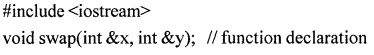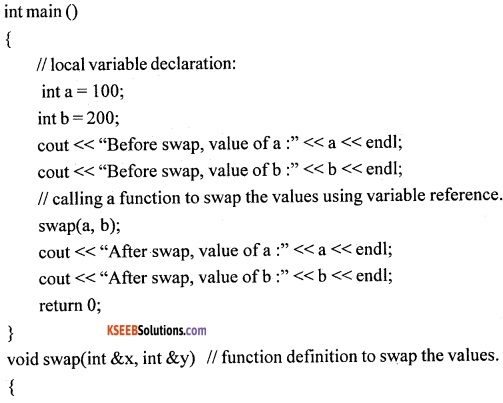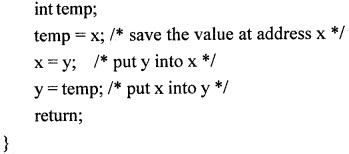# 1st PUC Computer Science Question Bank Chapter 13 User Defined Functions

You can Download Chapter 13 User Defined Functions Questions and Answers, Notes, 1st PUC Computer Science Question Bank with Answers Karnataka State Board Solutions help you to revise complete Syllabus and score more marks in your examinations.

## Karnataka 1st PUC Computer Science Question Bank Chapter 13 User Defined Functions

### 1st PUC Computer Science User Defined Functions One Mark Questions and Answers

Question 1.
What is a user defined function?
A user-defined FUNCTION is a self-contained block of statements that perform a specific task with a name given to it defined by the user in a program.

Question 2.
Mention any one advantage of user-defined function.
Code Reusability:
Writing the same sequence of code at two or more locations in the program can be avoided with the use of functions and universal use feature reduces rewriting of repetitive codes.

Question 3.
What is meant by function definition?
A function definition provides the actual body of the function with a name that you call it by, a list of zero or more arguments and it may give back a return value, of a particular type.Question 4.
What is a function declaration?
A function declaration tells the compiler about a function’s name, return type, and parameters.

Question 5.
What is meant by calling a function?
To use a function, user will have to call or invoke that function. It helps to execute the function and perform the process of a function.

Question 6.
What is a called function?
A called function is a function which is called for by the calling function.

Question 7.
How is a function invoked?
A function can be invoked by giving a call to that function.

Question 8.
Give the purpose of the return statement.
It is used to return the value from the called function to the calling function.

Question 9.
What is a function prototype?
The declaration of a function before it is used or called is known as function prototype.

Question 10.
Give the default return type of main() function.
The default return type of main() function is int data type.

Question 11.
What is a function parameter?
Sometimes the calling function supplies some values to the called function. These are known as function parameters.Question 12.
What is the significance of void in function return type?
A function that does not return a value is declared and defined as void.

Question 13.
Why are arguments used in functions?
Arguments are the mechanism that carries values from calling function to called function.

Question 14.
What are the actual arguments?
The data that is passed by the calling function as arguments/parameters is known as actual arguments, i.e., the arguments which are present at the time of function call.

Question 15.
What are formal arguments?
Formal arguments are the names of the arguments/parameters in the function header of called function.

Question 16.
What is a global variable?
A variable that is declared outside any function is known as a global variable.

Question 17.
What is the scope of a global variable?
The scope of a global variable is extended till the end of the program.

Question 18.
What are local variables?
The variables declared within a function block are called local variables.Question 19.
What is the use of a return statement in a function?
The return statement sends both control and value back to the calling function.

Question 20.
Mention the limitation of return statement.
The return statement return value is limited to a single value.

Question 21.
Write the syntax of the function prototype.
returntypespecifier function name( datatype argument1, datatype argument2, ..);

Question 22.
What is meant by scope of variables?
The scope of variable refers to the availability of variables for the use in different blocks of the program.

Question 23.
How are functions classified?
The Functions are classified as the following:-

• Functions with no argument and with no return value.
• Functions with argument and with no return value.
• Functions with no argument and with return value.
• Functions with argument and with return value.
• Recursive functions.

Question 24.
What are the different methods of calling a function?
The function can be called using either of the following methods:

1. call by value
2. call by reference

Question 25.
What are default arguments?
While defining a function, one can specify a default value for each of the arguments is called default arguments.

Question 26.
What is the use of default arguments?
The default value will be used if the corresponding argument is left blank when calling the function.Question 27.
What are the different types of arguments?

1. Actual arguments and
2. Formal arguments.

Question 28.
Mention the use of default arguments.
The default arguments are useful in a function where some arguments always have the same value.

Question 29.
What are constant arguments?
The arguments which are declared using a const keyword are called constant arguments.

Question 30.
What is the use of constant argument?
The value of a constant argument cannot be modified or changed during the execution of the function.

Question 31.
What is call/pass by value method of function call?
Pass by value or Call by value is the method where a copy of the value of the actual argument are passed to the called function to do a specific operation to return the result.

Question 32.
What is call by reference method of function call?
Pass by reference or call by reference is the method by which the addresses of the actual arguments are passed to the called function.Question 33.
How are arrays passed as arguments in functions?
The Arrays are passed as arguments in functions by referring to the name of the array.

Question 34.
How are structures passed as arguments in functions?
The Structures are passed to functions using pass by value method.

### 1st PUC Computer Science User Defined Functions Two/Three Marks Questions and Answers

Question 1.
What is the difference between in-built functions and user-defined functions?
A programmer has to write a user-defined function, whereas in-built functions are available to a programmer in the standard ‘c++’ libraries. Due to this, the in-built functions are also known as library functions.
Eg: sqrt(), setw(), strlen( ), strcat(), etc.,

Question 2.
Explain any three properties of functions.

1. It performs some well-defined task, which will be useful to other parts of the program.
2. The rest of the program doesn’t t have to know the details of how the function is implemented. This can make the rest of the program easier to think about.
3. One part of the program can be rewritten, to improve performance or add a new feature (or simply to fix a bug), without having to rewrite the rest of the program.

Question 3.
Explain any two advantages of functions.
1. Code Reusability:
Writing the same sequence of code at two or more locations in the program can be avoided with the use of functions and the Universal use feature reduces rewriting of codes.

2. Teamwork:
Functions promote teamwork. It divides a large bulky program into functionally independent modules and each person in the team can develop a separate subprogram.Question 4.
Write the structure of a function.
Function_type   function_name (data-type arg 1, data-type arg 2, … data-type arg n)
{
variable declarations;
statement 1;
statement 2;
……..
statement n;
retum(expression);
}

Question 5.
Explain function call with an example.
In the below example, the function demo is called by using the name of the function ‘demo’ as a statement of any calling function. t and r are the actual arguments of the function. It helps to execute the function and perform the process of a function.
Eg: demo(p,t,r);

Question 6.
What are the calling function and called function?
1. Calling function:
A function from which call to a function is given to another function is called a calling function.
For example, calling function is main() and called function is demo();

2. Called function:
A function which is called from another function.
For example, main () is calling function and demo(p,t,r) is the called function.

Question 7.
What are the actual parameters (actual arguments) and formal parameters (formal arguments)?
1. Actual parameters:
The data that are passed by the calling function as arguments/parameters are known as actual arguments, i.e., the arguments which are present at the time of function call.

2. Formal parameters:
There are the names of the arguments /parameters in the function header of the called function. Formal parameter values are used by the called function body.Question 8.
Explain local variables and global variables.
1. Local (internal) Variables:
The variables declared within a function block are called local variables. A variable can be used only within the function, where it is defined in Such a variable is accessible only from the function or block in which it is declared.

2. Global Variables:
They are the variables that are declared outside of main () function body or any other functional block. These variables can be used by all the modules and functions in the program.

Question 9.
What is a function prototype? Give an example.
The declaration of a function before it is used or called is known as function prototype.
Eg: int demo (int, int, int);

Question 10.
How are functions classified? Give the different types of functions.
A function depending upon whether arguments are passed or not and whether a value is returned or not can be classified into the following five categories.

• Functions with no arguments and no return value
• Functions with no arguments and with return value
• Functions with arguments and no return value.
• Function with arguments and return value.
• Recursive functions.

Question 11.
Give the syntax of function with no arguments and with no return values.
Syntax: void functionname()
{
statements;
}

Question 12.
Give the syntax of function with arguments and with no return values.
Syntax: void functionname(datatype,datatype)
{
statements;
}

Question 13.
Give the syntax of function with no arguments and with return values.
Syntax: int functionname()
{
statements;
retum(1);
}Question 14.
Give the syntax of function with arguments and with return values.
Syntax: int functionname(int, int)
{
statements;
retum(x);
}

### 1st PUC Computer Science User Defined Functions Five Marks Questions and Answers

Question 1.
Explain the various advantages of functions.
1. Modularity:
Functions can be used to divide a large bulky program into functionally independent modules or subprograms.

2. Universal use:
User-defined functions can be made a part of a library, which in turn, facilitates the usage of that function across other ‘C++’ programs.

3. Code Reusability:
Writing the same sequence of code at .two or more locations in the program can be avoided with the use of functions and universal use feature reduces repeated rewriting of codes.

4. Teamwork:
Functions promote teamwork. They Divide a large bulky program into functionally independent modules and each person in the team can develop a separate subprogram.

5. Reduction in program size:
Reduces the overall program size.

6. Easy Debugging:
Easy to detect errors and correct them in a module.Question 2.
Explain the structure of a function.
Function type function name (data-type arg1, data-type arg2, … data-type argn)
{
variable declarations;
statement 1;
statement 2;
……..
statement n;
return(expression);
}
1. Function_type:
The function-type identifies the type of return value, which will be sent back after the function has performed its task.
Eg: function type may be an int, float, char, void.

2. Function_name:
Function name followed by a pair of open and close parentheses ‘()’ helps to identify the name of the function and its arguments.
() The parentheses can contain an optional list of arguments that are passed to the function. Arguments are used to transfer/copy (pass) the values from calling function to called function.

3. Body of the function:
It is enclosed within braces. A function should have at least one executable statement and rest of the things are same as in main( ) function. The body of the function can also have an optional declaration for the variables to be used by the function only also called as local variables.

4. Return(expression):
The return statement can return the value of any expression. The return statement can contain an optional expression or value that is to be returned back to the calling function.

Question 3.
Write a short note on the scope of variables.
A scope is a region of the program and there are three places where variables can be declared:

• Inside a function or a block which are called local variables,
• Outside of all functions which are called global variables.

1. Local variables:
Variables that are declared inside a function or block are local variables. They can be used only by statements that are inside that function or block of code. Local variables are not known to functions outside their own.

2. Global variables:
Global variables are defined outside of all the functions, usually on top of the program. The global variables will hold their value throughout the lifetime of a program. A global variable can be accessed by any function. That is, a global variable is available for use throughout the entire program after its declaration.

It should be noted that a program pan have the same name for local and global variables but the value of a local variable inside a function will take preference.
For example,
#include <iostream>
int g_value = 0;
int main()
{
++g_value;
cout<< g_value <<end1;
int g_value= 100;
cout<< “g_value local variable which hides the global one: “ << g_value << end1;
cout<< “g_value global variable: “ << ::g_value << end1; /* In case we have a local variable with the same name as a global one, we can access the global variable using the global scope operator. */
return 0;
}
In the above example, we have declared a global variable, g_value, at the beginning of our program, outside any other block. As you can see, we can increment it in the function main and print its value to the screen.

The variables a, b and g value are local variables in the above program. The g_value variable is present in main() also. Now this variable g_value is a local to main() function which will hide the global variable. We can then access the local variable by its name, and as you can see it hides the global variable. Suppose we have to access global variable then we have to use the global scope operator,::, before the variable’s name: ::g_value.Question 4.
Explain functions with no argument and no return value with an example.
In the care of functions, where calling function gives function call to called function without any parameters, then called function processes statements of its own and returns back without any value to the calling function. Such called function returns type is normally declared with void data type.
For example,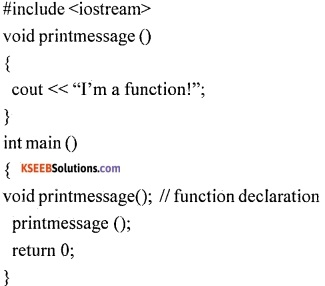In the above program, the main() and printmessage() which is a user defined function are the two functions. The function call is given by main() and called function printmessage() display message “ I’m a function” without returning any value.

The return type of printmessage() is void because function has no argument and no return value. The user-defined function printmessage() is defined with empty parentheses indicates the function is without any parameter. Such type of functions are called a function with no argument and no return value.

Question 5.
Explain functions with argument and no return value with an example.
The calling function main() gives the function call to the called function by passing arguments or values. Then the called function takes the values and performs the calculations and gives the output in itself but no value will be sent back to the calling function.
For example,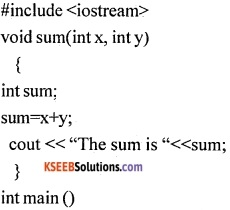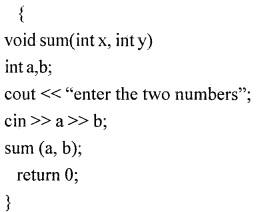In the above example, sum() is a user defined function. main() is a calling function gives a function call sum (a, b); with actual arguments a, b. The copy of values of a and b are sent to formal arguments x and y in the called function sum(). The function sum() performs addition and gives the result on the screen. Here no value being sent back to the calling function main() can be observed. These kinds of functions are called “Function with argument but no return values”.

Question 6.
Explain Functions with argument and with return value.
The calling function gives a function call to the called function by passing arguments or values. Then the called function takes those values and performs the calculations and returns a value, which will be sent back to the calling function. This is done using the return statement.
For example,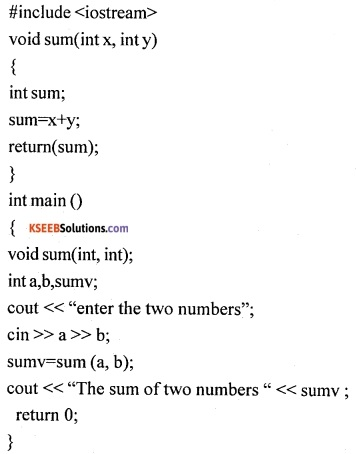In the above example, sum() is a user defined function void sum(int x, ini y)having two arguments. The statement int a,b,sumv; declare local variables in main() function. The statement sumv=sum (a, b); is a function call and actual arguments a and b values are sent to formal arguments x and y which is the local variable to sum() function. The statement return(sum); takes the control back along with the value present in the sum variable and assign it to the sumv variable in the calling function main(). This is how the function with argument and with return value works.Question 7.
Explain the working of function with no arguments and with return values.
In this type of function, the calling function gives the function call to the called function without sending any values. Then called function execute its own statements and return back to the calling function with a return value.
For example,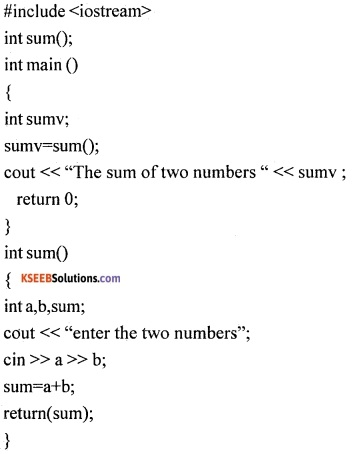In the above example, sum() is a user defined function. The calling function is main() gives the function call without passing any argument sumv=sum(); and called function sum() accept the values and gives out result back to calling function. The main() function displays the output.

Question 8.
Explain passing default argument to the function, with an example.
When we define a function we can specify a default value for each of the parameters. This value will be used if the corresponding argument is left blank during function call to the called function. This is done by using the assignment operator and assigning values for the arguments in the function definition.

During the function call, if a value for that parameter is not passed then a default value is used, but if a value is mentioned then this default value is ignored and the passed value is used.
Consider the following example: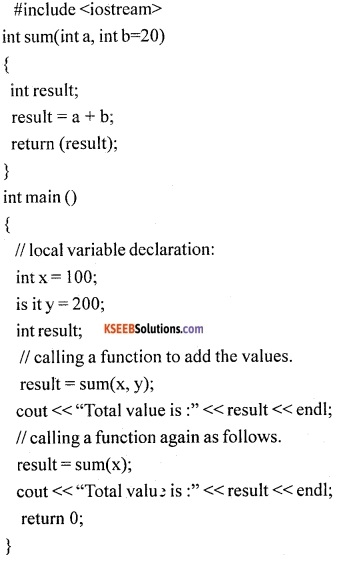In the above program, the statement int sum(int a, int b=20) in the function header indicate the default argument tc the variable b is assigned with the value 20. During the first function call, the statement result = sum(x, y); copies the value of x and y to the called function and processes the result.

During the second-time function call i.e., result = sum(x);, only one argument is mentioned and the value for the second argument is obtained from the default argument which is mentioned in the function definition i.e., b=20 and gives the processed result.Question 9.
Explain function call using pass by value technique with an example.
In pass by value mechanism, during the function call actual arguments send the copy of values to formal arguments that are present in called function. These formal arguments are copies of the actual arguments and are stored in the temporary locations of the memory. During the process by the called function, the changes made to the formal arguments do not change the actual arguments.
For example,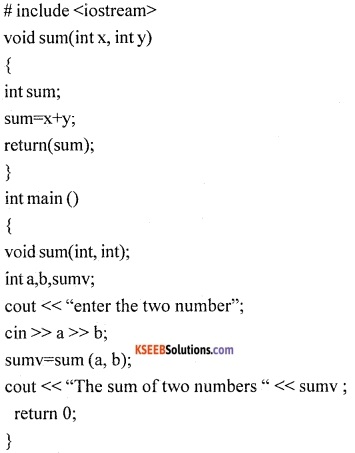In the above example, sum() is a user defined function void sum(int x, int y)having two arguments. The statement int a,b,sumv; declare local variables in main() function. The statement sumv=sum (a, b); is a function call and actual arguments a and b values are sent to formal arguments x and y which is the local variable to sum() function. The statement return(sum); takes the control back along with the value present in the sum variable and assign it to the sumv variable in the calling function main().

Question 10.
Explain function call using pass by reference technique with an example.